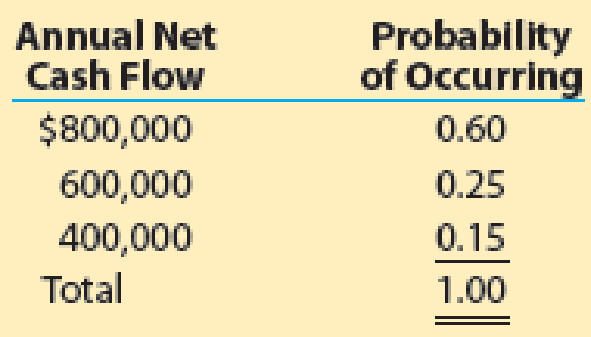# Assume Boulder Creek Industries in MAD 26-3 assigns the following probabilities to the estimated annual net cash flows: a. Compute the expected value of the annual net cash flows. b. Determine the expected net present value of the equipment, assuming a desired rate of return of 12% and expected annual net cash flows computed in part (a). Use the present value tables (Exhibits 2 and 5) provided in the chapter in determining your answer. c. Based on your results in parts (a) and (b), should Boulder Creek Industries invest in the equipment?### Financial And Managerial Accounting

15th Edition
WARREN + 1 other
Publisher: Cengage Learning,
ISBN: 9781337902663

#### Solutions

Chapter
Section### Financial And Managerial Accounting

15th Edition
WARREN + 1 other
Publisher: Cengage Learning,
ISBN: 9781337902663
Textbook Problem
1 views

## Assume Boulder Creek Industries in MAD 26-3 assigns the following probabilities to the estimated annual net cash flows:a. Compute the expected value of the annual net cash flows. b. Determine the expected net present value of the equipment, assuming a desired rate of return of 12% and expected annual net cash flows computed in part (a). Use the present value tables (Exhibits 2 and 5) provided in the chapter in determining your answer. c.Based on your results in parts (a) and (b), should Boulder Creek Industries invest in the equipment?

a.

To determine

Compute the expected value of the annual net cash flows.

### Explanation of Solution

Compute the expected value of the annual net cash flows:

 Annual net cash flow Probability of occurring Expected Value $800,000 0.60$480,000 \$600,000 0...

b.

To determine

Compute the net present value of the equipment, assuming 12% desired rate of return for the given annual net cash flows in part (a).

c.

To determine

Recommend whether Company BC should invest in the equipment based upon parts (a) and (b).

### Still sussing out bartleby?

Check out a sample textbook solution.

See a sample solution

#### The Solution to Your Study Problems

Bartleby provides explanations to thousands of textbook problems written by our experts, many with advanced degrees!

Get Started

Find more solutions based on key concepts
What is an embedded audit module?

Accounting Information Systems

What steps are followed in posting cash receipts from the general journal to the general ledger?

College Accounting, Chapters 1-27 (New in Accounting from Heintz and Parry)

Would it ever be rational for a firm to borrow money in order to pay cash dividends? Explain.

Fundamentals of Financial Management, Concise Edition (with Thomson ONE - Business School Edition, 1 term (6 months) Printed Access Card) (MindTap Course List)